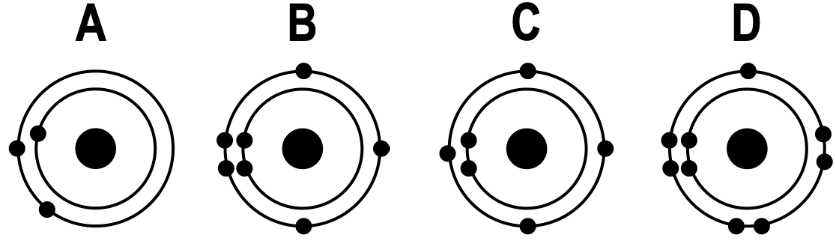## Microbiology

Learn the toughest concepts covered in Microbiology with step-by-step video tutorials and practice problems by world-class tutors.

3. Chemical Principles of Microbiology

# Ionic Bonding

1
concept

## Ionic Bonding4m
Play a video:
in this video, we're going to begin our lesson on ionic bonding. But before we could talk about ionic bonding, we first need to be able to understand what ions are, and we need to be able to distinguish between an ions and cat ions. And so ions is really just a general term that refers to atoms or molecules with a net electrical charge. Now the charge on an ion can either be a negative or a positive charge due to either the gain or the loss of negatively charged electrons. And so, really, this is what leads to the two types of ions, which are once again and ions and cat ions. And so an ions, as their name implies with so many ends in their name, are going to be negatively charged ions. And so these negatively charged and ions air going to result from the gain of a negatively charged electron. And so, of course, if an Adam gains a negatively charged electron, then it can become and an eye on a negatively charged ion. Now, on the other hand, cat ions, um, as their name implies with the tea here, um, are going to be positively charged, and so you can think the tea is for the plus sign. That means positively charged. So cat ions are positively charged ions that result from, of course, the loss of a negatively charged electron. And so, if in Adam gives up something a negatively charged electron, then itself it's going to become more positive. It's gonna become more positive itself. And so if we take a look at our example image down below, weaken further, distinguish between an ions and cat ion. So notice here in the center of our image, what we're showing you is a single, uh, neutral hydrogen atom. And it is neutral because hydrogen atoms are characterized by having just one proton in their nucleus and notice that it also has one electron here in this middle image. And so because it has one electron and one proton and its nucleus, those two charges cancel each other out. And what we get is a neutral hydrogen atom right here in the middle. Now, if this neutral hydrogen atom were to gain a negatively charged electron like this one right here so that it now has two electrons instead of just one like it did before. Uh, then it's gonna have one more electron than Proton and that's going to give it an overall net negative charge. Like what we see here. And so this is what's going to make it an an ion. Once again, you can thank all of these ends here. The two ends in an ion suggest that it is negatively charged. Now, on the other hand, if we were to take this neutral hydrogen atom here in the center and this time we were to lose the electron, if there was a loss of the electron on that electron were transferred to something else, then all we would have is ah, hydrogen atom with just a single proton in the nucleus and it would not have any electrons. And so there would be a positive charge on this hydrogen atom. And so this is what makes it a cat ion. And so you can think that once again, the tea here in Cat Ion is for the plus sign and positive charge. And so really, that's the biggest difference here between an ions and cat. Ions and ions are negatively charged, whereas cat ions are positively charged. And if We're just saying the term ion. Then it could either be an an ion or a cat ion. So we would have to further distinguish the ion too. Determine what it iss Uh, but for now, this here concludes our introduction to ions and ions versus cat ions, and we'll be able to talk about the Ionic bonding as we move forward. So I'll see you all in our next video.
2
Problem

When atoms gain or lose electrons, they become negatively or positively charged. They are known as:

3
Problem

Which of the following statements is true of ALL atoms that are anions?

4
Problem

If oxygen has 9 electrons it will be a ______________________:

5
concept

## Ionic Bonds6m
Play a video:
6
Problem

An ionic bond is a bond in which:

7
Problem

Cations and anions would be most frequently associated with which of the following:

8
Problem

When are atoms most stable?

9
Problem

Which of the following neutral atoms would be most likely to lose an electron and become a cation of +1?